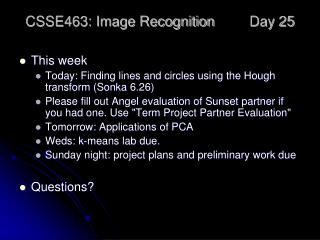DownloadDownload PresentationCSSE463: Image Recognition Day 25

# CSSE463: Image Recognition Day 25

Download Presentation## CSSE463: Image Recognition Day 25

- - - - - - - - - - - - - - - - - - - - - - - - - - - E N D - - - - - - - - - - - - - - - - - - - - - - - - - - -
##### Presentation Transcript

1. CSSE463: Image Recognition Day 25 • This week • Today: Finding lines and circles using the Hough transform (Sonka 6.26) • Please fill out Angel evaluation of Sunset partner if you had one. Use "Term Project Partner Evaluation" • Tomorrow: Applications of PCA • Weds: k-means lab due. • Sunday night: project plans and preliminary work due • Questions?

2. Finding lines in real images • Input: set of edge points • Output: the equation of a line containing them • Methods: • Least-squares (if you know which points belong to the line…) • Hough transform (today)

3. Hough transform • Idea (Sonka 6.2.6; Forsyth and Ponce, ch 15): • Represent a line using parameters • Each edge point in the image casts a vote for all lines of which it could be part. • Only the true line receives lots of votes

4. Parametric Equation of a Line • Represent a line using 2 parameters • y = mx + b? • Problem? • Ax + By + C = 0? • 3 parameters; but A, B, and C are related…we only need 2 • r and q • r is distance from line to origin • Q is the angle the distance segment makes with x-axis • x cosq + y sinq = r Q1

5. Voting • Each point in image votes for all lines of which it could be part. • Only “true” line receives lots of votes. • Quiz question: show (4,4), (2,2), and (0,0) voting for a line in y = mx+b space (for simplicity) Q2-3

6. Perfect line • Notice sharp peak in voting space • (next 3 images from Forsyth and Ponce, ch 15) Q4

7. Approximate line • Notice the broader peak. Can we detect it? • Could smooth or use a coarser quantization? • Accumulator array: bin size? Range? Q5

8. Random noise • Votes spead all over the place: no line • Too much noise creates “phantom lines” • Smoothing can sometimes help Q6

9. Limitations • Finding the right grid size in parameter space may be tricky • Trial and error

10. Matlab • Run an edge detector first to find points that are voting • [H, theta, rho] = hough(edgeImg); • peaks = houghpeaks(H,nPeaks); • This works for lines only

11. Another demo http://www.rob.cs.tu-bs.de/content/04-teaching/06-interactive/HNF.html

12. Generalizations • Finding circles with fixed radius… • Finding circles with arbitrary radius… • Finding line segments • Finding arbitrary shapes… • Ballard, Dana. 1981. Generalizing the Hough transform to detect arbitrary shapes. Pattern Recognition, 13(2):111-122. • Dana was a long-time member of Rochester’s computer vision group. Q7-8

13. My Circle Finder • Demo • Wouldn’t this be a great lab? • Like Matlab’shough and houghpeaks (for lines), but from scratch • Easier would be to find circles of fixed radius

14. Reducing the number of votes • Use the edge gradient information as well • Only need to cast votes for centers along the gradient • I’ve done this; it works really well • Use partial curves. If you had a way of grouping relating points, you could use curvature. • Haven’t done yet.